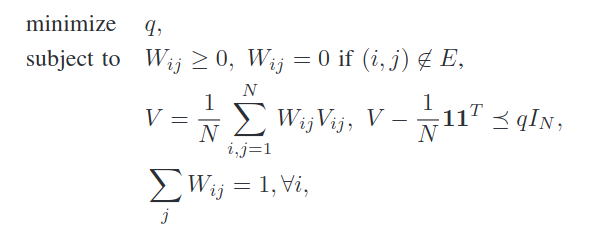How to write the product of CVX variables in a convex way?

Hello All,

I am trying to code the semi-definite optimization problem which is shown in the picture using CVX.in the picture above, (i,j) not in E means that node i and j are neighbors.
I wrote the following code so far (this is what I could do so far. It definitely needs improvement.)

cvx_begin sdp
agt = struct([]);
N = 2;
for i = 1:N
agt(i).neighbors = neighbors{i};
end
variable q
variable W(N,N) symmetric
variable V(N,N) symmetric
subject to
minimize (q)
j = 1;
for i = 1:N
W(i,j)>=0;
j = j+1;
end

j = 1;
for i = 1:N
D =[i,j];
if ~ismember(D,agt(i).neighbors)
W(i,j)=0;
end
j = j+1;
end

dummy = 0;

for i = 1:N
for j = 1:N
dummy = W(i,j)*V(i,j);
V  = (1/N)*(dummy + V);
end
end

V-(1/N)*ones(N,1)*ones(1,N) - q*eye(N) == semidefinite(N);

for k = 1 : N
sum( diag( X, k ) ) == 1;
end

cvx_end

When I run this code, I get the error

Error in * (line 36)
z = feval( oper, x, y );

Error in lambda (line 31)
dummy = W(i,j)*V(i,j);

From what I read, we can not use the product of two CVX variables. My main question is about how to resolve this error.
If there is any other suggestions on the rest if code, I greatly appreciate since I am new to CVX.

Thanks!

That is very confusing notation (at least without the benefit of seeing the preceding or explanatory material in the paper or book). It looks like each V_{ij} is supposed to be a matrix, and constitutes input data, not an optimization variable; yet W_{ij} is an element of a matrix W. Then V is an expression (which uses =, not == to construct) in terms of W and all the V_{ij} matrices. With this interpretation, V is affine, and the formation of V, as well as the semidefinite constraint it is used in, would be accepted by CVX. You need to check whether this interpretation is correct.

There are other issues with your code: A non-exhaustive list includes W_{ij} \ge 0 constraint does not capture all elements, and can simply be written W(:) >= 0. Constraint on certain elements of W being zero should be W(i,j) == 0, not W(i,j) = 0. There may be plenty more which is wrong.

I suggest you carefully re-read the entirety of the CVX Users Guide, and start with some problems not having the complicated indexing. And of course you need to understand the paper or book you are extracting a model from.

Thank you so much! Unfortunately, there is not much explanation regarding the optimization part in the source I have. This problem can be found on page 5 of this paper. .
Interestingly, the main source of this problem is from a paper by Dr. S Boyd himself. On page 10 (Eq 53) of this paper, this optimization problem is mentioned.

I will read more about the points you have mentioned. Thanks again!

Mr. Stone, I was wondering if you can reply to this question.
So, with the interpretation that V_{ij} is a matrix and V is an expression, I declared V and V_{ij} like this:

variable V_ij(N,N) symmetric
expression V

And I wrote the constraint V=1/N\sum^{n}_{i,j=1}W_{ij}V_{ij} like this:

for i = 1:N
for j = 1:N
V = W(i,j).*V_ij;
end
end
V = (1/N).*V;

I still get the same error. I would greatly appreciate it if you could help me with this.

I believe there are N^2 matrices V_{ij} of input data, as well as V being an input matrix of the same dimensions as the V_{ij}.

Therefore, place the V_{ij} in a 4D array, V_array with the first two dimensions being those of each V_{ij}, and the last two dimensions being N and N. V_array is input data and must be fully populated before use in CVX. As well, V.

cvx_begin
variable q
variable W(N,N) % not symmetric as you may have done somewhere
minimize(q)
W(:) >= 0
% Add W(i,j) == 0 constraints  for i,j not in E (left to you)
weighted_sum = 0;
for i=1:N
for j=1:N
weighted-sum = weighted_sum + W(i,j)*V_array(:,:,i,j);
end
end
V == weighted_sum/N
-(V - 1/N*ones(N,N) -q*eye(N)) == semidefinite(N)
sum(W,2) == 1
cvx_end

Edited comment line to make clear that constraints to be added for W(i,j) == 0 need to use ==.

Of course, the input data, V_array and V must be appropriately chosen, or the problem won’t even be feasible.

Mr. Stone, Thank you so much!

I just corrected my code to make the LHS of the semidefinite constraint the negative of what it was. The edited version of the post should now be correct.

On 2nd thought, I’m not sure whether V is input data, or just an expression formed from the RHS. If the latter, the only change to the code is to use V = instead of V == . But that is for you to figure out.

I really appreciate your consideration! Actually, this has kept me confused and thinking since yesterday. That is why I am reading the source paper very carefully one more time.